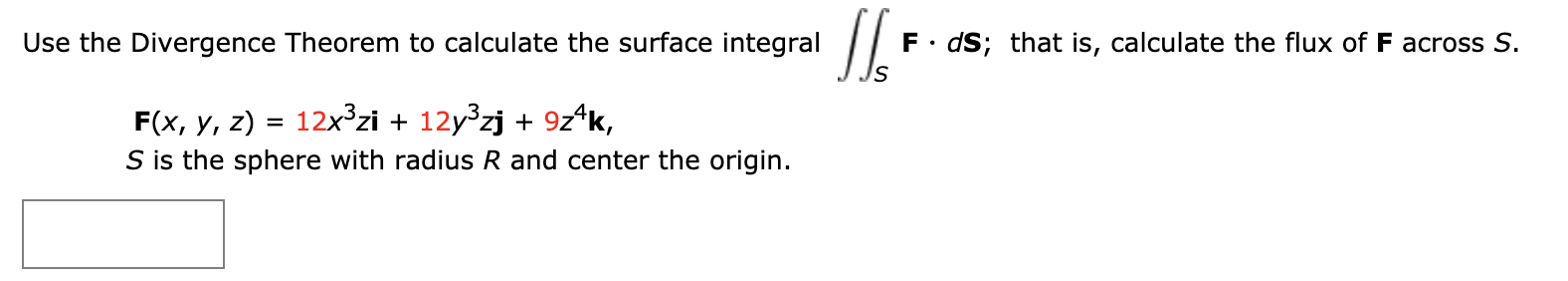# П. F• dS; that is, calculate the flux of F across S. Use the Divergence Theorem to calculate the surface integral 12x3zi + 12y zj + 9z^k, F(x, y, z) S is the sphere with radius R and center the origin.

Question

Use the Divergence Theorem to calculate the surface integral

 S
F · dS;

that is, calculate the flux of F across S.

F(x, y, z) = 12x3zi + 12y3zj + 9z4k,

S is the sphere with radius R and center the origin.help_outlineImage TranscriptioncloseП. F• dS; that is, calculate the flux of F across S. Use the Divergence Theorem to calculate the surface integral 12x3zi + 12y zj + 9z^k, F(x, y, z) S is the sphere with radius R and center the origin. fullscreen